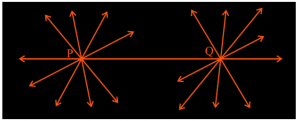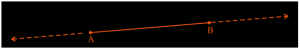# Introduction To Euclidean Geometry

Euclidean geometry is the study of geometrical shapes and figures based on different axioms and theorems. It is basically introduced for flat surfaces. It is better explained especially for the shapes of geometrical figures and planes. This part of geometry was employed by Greek mathematician Euclid, who has also described it in his book, Elements. Therefore this geometry is also called Euclid geometry

The axioms or postulates are the assumptions which are obvious universal truths, they are not proved. Euclid has introduced the geometry fundamentals like geometric shapes and figures in his book elements and has stated 5 main axioms or postulates. Here, we are going to discuss the definition of euclidean geometry, its elements, axioms and five important postulates.

## History of Euclid Geometry

The excavations at Harappa and Mohenjo-Daro depict the extremely well-planned towns of Indus Valley Civilization (about 3300-1300 BC). The flawless construction of Pyramids by the Egyptians is yet another example of extensive use of geometrical techniques used by the people back then. In India, the Sulba Sutras, textbooks on Geometry depict that the Indian Vedic Period had a tradition of Geometry.

The development of geometry was taking place gradually, when Euclid, a teacher of mathematics, at Alexandria in Egypt, collected most of these evolutions in geometry and compiled it into his famous treatise, which he named ‘Elements’.

 Plane geometry Congruence of triangles Similarity of triangles Areas Pythagorean theorem Circles Regular polygons Conic sections Solid geometry Volume Regular solids

## What is Euclidean Geometry?

Euclidean Geometry is considered as an axiomatic system, where all the theorems are derived from the small number of simple axioms. Since the term “Geometry” deals with things like points, line, angles, square, triangle, and other shapes, the Euclidean Geometry is also known as the “plane geometry”. It deals with the properties and relationship between all the things.

Non-Euclidean is different from Euclidean geometry. There is a difference between these two in the nature of parallel lines. In Euclid geometry, for the given point and line, there is exactly a single line that passes through the given points in the same plane and it never intersects.

## Euclid’s Elements

Euclid’s Elements is a mathematical and geometrical work consisting of 13 books written by ancient Greek mathematician Euclid in Alexandria, Ptolemaic Egypt. Further, the ‘Elements’ was divided into thirteen books which popularized geometry all over the world. As a whole, these Elements is a collection of definitions, postulates (axioms), propositions (theorems and constructions), and mathematical proofs of the propositions.

Book 1 to 4th and 6th discuss plane geometry. He gave five postulates for plane geometry known as Euclid’s Postulates and the geometry is known as Euclidean geometry. It was through his works, we have a collective source for learning geometry; it lays the foundation for geometry as we know now.

## Euclidean Axioms

Here are the seven axioms given by Euclid for geometry.

1. Things which are equal to the same thing are equal to one another.
2. If equals are added to equals, the wholes are equal.
3. If equals are subtracted from equals, the remainders are equal.
4. Things which coincide with one another are equal to one another.
5. The whole is greater than the part.
6. Things which are double of the same things are equal to one another.
7. Things which are halves of the same things are equal to one another

## Euclid’s Five Postulates

Before discussing Euclid’s Postulates let us discuss a few terms as listed by Euclid in his book 1 of the ‘Elements’. The postulated statements of these are:

• Assume the three steps from solids to points as solids-surface-lines-points. In each step, one dimension is lost.
• A solid has 3 dimensions, the surface has 2, the line has 1 and point is dimensionless.
• A point is anything that has no part, a breadthless length is a line and the ends of a line point.
• A surface is something which has length and breadth only.

It can be seen that the definition of a few terms needs extra specification. Now let us discuss these Postulates in detail.

### Euclid’s Postulate 1

“A straight line can be drawn from anyone point to another point.”

This postulate states that at least one straight line passes through two distinct points but he did not mention that there cannot be more than one such line. Although throughout his work he has assumed there exists only a unique line passing through two points.### Euclid’s Postulate 2

“A terminated line can be further produced indefinitely.”

In simple words what we call a line segment was defined as a terminated line by Euclid. Therefore this postulate means that we can extend a terminated line or a line segment in either direction to form a line. In the figure given below, the line segment AB can be extended as shown to form a line.### Euclid’s Postulate 3

“A circle can be drawn with any centre and any radius.”

Any circle can be drawn from the end or start point of a circle and the diameter of the circle will be the length of the line segment.

### Euclid’s Postulate 4

“All right angles are equal to one another.”

All the right angles (i.e. angles whose measure is 90°) are always congruent to each other i.e. they are equal irrespective of the length of the sides or their orientations.

### Euclid’s Postulate 5

“If a straight line falling on two other straight lines makes the interior angles on the same side of it taken together less than two right angles, then the two straight lines, if produced indefinitely, meet on the side on which the sum of angles is less than two right angles.”

Further, these Postulates and axioms were used by him to prove other geometrical concepts using deductive reasoning. No doubt the foundation of present-day geometry was laid by him and his book the ‘Elements’.

### Euclid Geometry Worksheet

1. How many dimensions do solids, points and surfaces have?
2. What is the shape of a pyramid’s base?
3. If a + b =10 and a = c, then prove that c + b =10.
4. Can two distinct intersecting line be parallel to each other at the same time? Justify.
5. Read the following sentence and mention which of Euclid’s axiom is followed: “X’s salary is equal to Y’s salary. Due to the recession, the salaries of X and y are reduced to half. Now the final salary of X will still be equal to Y.”

So here we had a detailed discussion about Euclid geometry and postulates. Keep visiting BYJU’S to get more such maths topics explained in an easy way. Also, register now and access numerous video lessons on different maths concepts.

## Frequently Asked Questions – FAQs

### What is Euclidean Geometry?

Euclidean geometry is the study of flat shapes or figures of flat surfaces and straight lines in two dimensions.

### What is the difference between Euclidean and non-Euclidean Geometry?

Euclidean geometry deals with figures of flat surfaces but all other figures which do not fall under this category comes under non-Euclidean geometry. For example, curved shape or spherical shape is a part of non-Euclidean geometry.

### What are the five postulates of Euclid’s geometry?

1. A straight line may be drawn from any point to another point.
2. A terminated line can be produced indefinitely.
3. A circle can be drawn with any centre and any radius.
4. All right angles are equal to each other.
5. If a straight line falls on two straight lines makes the interior angles on the same side of it taken together less than two right angles, then the two straight lines, if produced indefinitely, meet on that side on which the sum of angles is less than two right angles.

### What are the three types of geometry?

In two-dimensional plane, there are majorly three types of geometries.
Euclidean (for flat surfaces)
Spherical (for curved surfaces)
Hyperbolic

### What is the use of euclidean geometry?

Euclidean geometry is majorly used in the field of architecture to build a variety of structures and buildings. Designing is the huge application of this geometry. Also, in surveying, it is used to do the levelling of the ground.

### Mention three axioms given by Euclid.

1. Things which are equal to the same thing are equal to one another
2. The whole is greater than the part
3. Things which coincide with one another are equal to one another